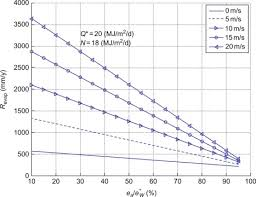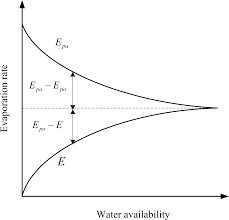## How to Calculate and Solve for Work of Evapouration (wet steam) | Enthalpy

The work of evapouration(wet steam) is illustrated by the image below.To compute for work of evapouration, three essential parameters are needed and these parameters are Specific Volume of Dry Gas (vg), Specific Volume of Wet Steam (vf), Pressure of Gas (P) and Dryness Fraction(x).

The formula for calculating work of evapouration:

WE = Px(vg – Vf)

Where:

WE = Work of Evapouration (Wet Steam)
vg = Specific Volume of Dry Gas
vf = Specific Volume of Wet Steam
P = Pressure of Gas
x = Dryness Fraction

Given an example;
Find the work of evapouration when the specific volume of dry gas is 12, the specific volume of wet steam is 0, the pressure of gas is 5 and the dryness fraction is 8.

This implies that;

vg = Specific Volume of Dry Gas = 12
vf = Specific Volume of Wet Steam = 0
P = Pressure of Gas = 5
x = Dryness Fraction = 8

WE = Px(vg – Vf)
WE = 5 x 8 x (12 – 0)
WE = 5 x 8 x 12
WE = 480

Therefore, the work of evapouration is 480J.

Calculating the Specific Volume of Dry Gas when the Work of Evapouration, the Specific Volume of Wet Steam and the Pressure of Gas is Given.

vg = WE / Px + vf

Where:

vg = Specific Volume of Dry Gas
WE = Work of Evapouration
vf = Specific Volume of Wet Steam
P = Pressure of Gas
x = Dryness Fraction

Given an example;
With work of evapouration as 40, the specific volume of wet steam as 0, the pressure of gas as 10 and the dryness fraction as 2. Find the specific volume of dry gas?

This implies that;

WE = Work of Evapouration = 40
vf = Specific Volume of Wet Steam = 0
P = Pressure of Gas = 10
x = Dryness Fraction = 2

vg = WE / Px + vf
vg = 40 / 10(2) + 0
vg = 40 / 20 + 0
vg = 2

Therefore, the specific volume of dry gas is 2.

## How to Calculate and Solve for Work of Evapouration | Enthalpy

The work of evapouration is illustrated by the image below.To compute for work of evapouration, three essential parameters are needed and these parameters are Specific Volume of Dry Gas (vg), Specific Volume of Wet Steam (vf) and Pressure of Gas (P).

The formula for calculating work of evapouration:

WE = P(vg – Vf)

Where:

WE = Work of Evapouration
vg = Specific Volume of Dry Gas
vf = Specific Volume of Wet Steam
P = Pressure of Gas

Given an example;
Find the work of evapouration when the specific volume of dry gas is 30, the specific volume of wet steam is 0 and the pressure of gas is 10.

This implies that;

vg = Specific Volume of Dry Gas = 30
vf = Specific Volume of Wet Steam = 0
P = Pressure of Gas = 10

WE = P(vg – Vf)
WE = 10 x (30 – 0)
WE = 10 x 30
WE = 300

Therefore, the work of evapouration is 300J.

Calculating the Specific Volume of Dry Gas when the Work of Evapouration, the Specific Volume of Wet Steam and the Pressure of Gas is Given.

vg = WE / P + vf

Where:

vg = Specific Volume of Dry Gas
WE = Work of Evapouration
vf = Specific Volume of Wet Steam
P = Pressure of Gas

Given an example;
With work of evapouration as 40, the specific volume of wet steam as 0 and the pressure of gas as 10. Find the specific volume of dry gas?

This implies that;

WE = Work of Evapouration = 40
vf = Specific Volume of Wet Steam = 0
P = Pressure of Gas = 10

vg = WE / P + vf
vg = 40 / 10 + 0
vg = 4

Therefore, the specific volume of dry gas is 4.